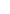## Passive Components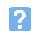1. Which one of the following is NOT a preferred value in the E6 series?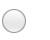a) 1.5b) 2.2c) 3.9d) 6.82. Which of the following gives the value of the resistor shown below?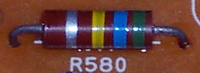a) 56 kohm, 5%b) 560 kohm, 10%c) 580 kohm, 5%d) 680 kohm, 10%.3. The component shown below is: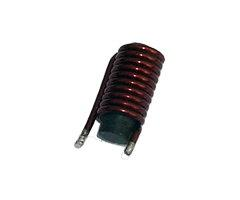a) an air-cored inductorb) a variable transformerc) an air-spaced capacitord) a ferrite-cored inductor.4. Which one of the following gives the effective resistance of the circuit shown below?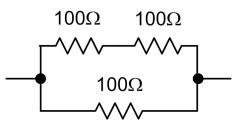a) 67 ohmb) 100 ohmc) 150 ohmd) 300 ohm.5. What type of component is shown below?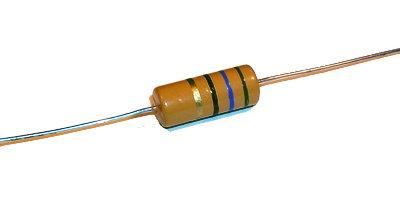a) Metal clad resistorb) Carbon film resistorc) Ceramic coated wrirewound resistord) Vitreous enamel coated wirewound resistor.6. A current of 2 A flows in a 5 H inductor. What energy is stored in the inductor?a) 2.5 Jb) 5 Jc) 10 Jd) 20 J.7. Which one of the symbols shown below represents an electrolytic capacitor?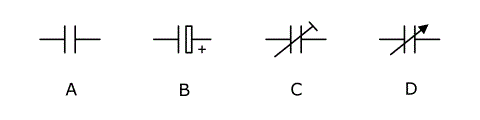a) Ab) Bc) Cd) D.8. The resistance of a light dependent resistor (LDR):a) is usually zero in total darknessb) falls as the light level increasesc) increases as the light level increasesd) remains constant over a wide range of light levels.9. The component shown below is: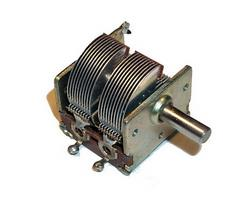a) a fixed inductorb) a variable inductorc) a fixed capacitord) a variable capacitor.10. A capacitor of 20 uF is charged to a potential of 50 V. Which one of the following gives the charge stored?a) 1 mCb) 2.5 uCc) 2.5 mCd) 400 uC.11. A ceramic capacitor is marked '102K'. Which one of the following gives its value?a) 1 nF, 10%b) 10 nF, 2%c) 102 nF, 10%d) 102 kF, 10%.12. The component shown below is: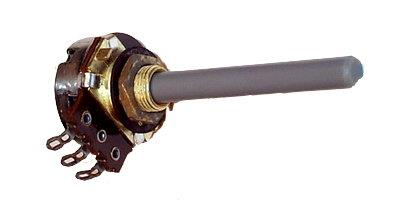a) a pre-set resistorb) a variable resistorc) a pre-set capacitord) a variable capacitor.13. The component shown below is: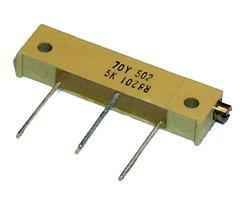a) a pre-set resistorb) a variable resistorc) a pre-set capacitord) a variable capacitor.14. Which one of the following types of capacitor has the highest stability?a) micab) ceramicc) polyesterd) electrolytic.15. Which one of the following types of resistor has the highest power rating?a) metal cladb) carbon rodc) carbon filmd) vitreous enamel coated wirewound.
This is more feedback!
This is the feedback!